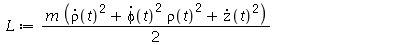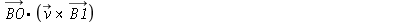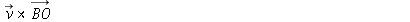# Question:evaluation/simplification of vector relations using the physics-package

## Question:evaluation/simplification of vector relations using the physics-package

Maple 17

Hello,

1) I would like to ask how I can force Maple to explicitly evaluate a summation over repeated indices. As an example: I would like to do a very simple thing, namely to evaluate the Lagrangian of a free particle in let's say cylindrical coordinates, as follows:

restart:
with(Physics):
with(Physics[Vectors]):
Setup(mathematicalnotation=true, dimension=3):
Coordinates(X=[rho,phi,z]);

Setup(metric = drho^2 + rho(t)^2*dphi^2 + dz^2, spacetimeindices=lowercaselatin_is);  # don't know whether rho  instead of rho(t) should be used here in order to use the metric consistently with the Coordinates-command...

print(g_[]);

Parameters(m);

L := m/2*g_[i,j].diff(X[~i](t),t).diff(X[~j](t),t);

Simplify(L);   # the result is not evaluated further

# I could easily do that manually by invoking

with(LinearAlgebra):
dot_q := map(diff, <x1(t),x2(t),x3(t)>, t);
L1 := Transpose(dot_q).(g_[].dot_q);

L2 := m/2*L1;

L := rhs(L2);   # which gives the desired result:Is it possible to get to that point without making a detour using the LinearAlgebra-package?

2)  The Physics[Vectors]-package very nicely recognizes vectors being orthogonal when performing dot products like

B_ . (v_ &x B_);

and gives the desired output. Yet when I just slightly modify this, let's say performing an expansion of a magnetic field

B0_ . (v_ &x (B0_ + B1_));

Simplify(%);

Maple is not able to simplify this to the desiredeven if I expand the second factor in parenthesis by myself, which is quite inconvenient for then I have to deleteby hand, maybe most quickly with the op-command. If one wants to, let's say, compute a high-order Larmor radius expansion this results in some really tedious work, although one could readily see which terms are zero due to orthogonality just by looking at the expressions. Did I probably miss some trick how to do this efficiently?